# How to use SUMIF in Excel - formula examples to conditionally sum cells

This tutorial explains the Excel SUMIF function in plain English and provides a numbers of SUMIF formula examples for numbers, text, dates and wildcards.

If you are faced with the task that requires conditional sum in Excel, the SUMIF function is what you need. This tutorial will briefly explain the function's syntax and general usage, and then we will apply the new knowledge in practice by making a few SUMIF formula examples.

A good thing is that the SUMIF function is identical in all Excel versions, from 2016 to 2003. Another great news is that once you've invested some time in learning SUMIF, it will take you very little effort to get the insight of other "IF" functions such as SUMIFS, COUNTIF, COUNTIFS, AVERAGEIF etc.

## SUMIF in Excel - syntax and usage

The SUMIF function, also known as Excel conditional sum, is used to add cells based on a certain condition, or criteria.

If you've happened to read the COUNTIF tutorial on this blog, you won't have any difficulties with understanding Excel SUMIF because its syntax and usage is analogous. So, the syntax of the SUMIF function is as follows:

SUMIF(range, criteria, [sum_range])

As you see, the SUMIF function has 3 arguments - first 2 are required and the 3rd one is optional.

1. `range` - the range of cells to be evaluated by your criteria, for example A1:A10.
2. `criteria` - the condition that must be met. The criteria may be supplied in the form of a number, text, date, logical expression, a cell reference, or another Excel function. For example, you can enter the criteria such as "5", "cherries", "10/25/2014", "<5", "A1", etc.
Note. Please pay attention that any text criteria or criteria containing mathematical symbols must be enclosed in double quotation marks ("). For numerical criteria, double quotation marks are not required.
3. `sum_range` - the cells to sum if the condition is met. This argument is optional, and you need to use it only if you want to sum cells other than defined in the range argument. If the sum_range argument is omitted, Excel will sum the same cells to which the criteria is applied (i.e. specified in the range argument).

To illustrate the Excel SUMIF syntax better, let's consider the following example. Suppose you have a list of products in column A and corresponding amounts in column C. You want to know the sum of all amounts relating to a given product, e.g. bananas.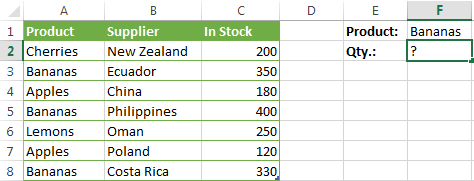Now, let's define the arguments for our SUMIF formula:

• range: A2:A8
• criteria: "bananas"
• sum_range: C2:C8

Putting this together, you get: `=SUMIF(A2:A8, "bananas", C2:C8)`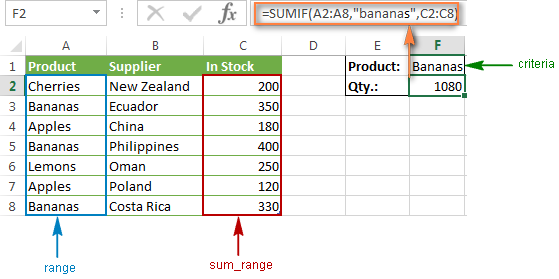This formula example demonstrates the simplest usage of the SUMIF function with the text criteria. Instead of text, you can include a number, date or a cell references in your criteria. For instance, you can re-write the above formula so that it references the cell containing the name of the product to be summed:

`=SUMIF(A2:A8,F1,C2:C8)`

Note. The sum_range parameter actually specifies only the upper leftmost cell of the range to be summed. The remaining area is defined by the dimensions of the range argument.

In practice, this means that the sum_range argument does not necessarily have to be of the same size as the range argument, i. e. it may have a different number of rows and columns. However, the first cell (i.e. the top left cell) of the sum range must always be the right one. For example, in the above SUMIF formula, you can supply C2, or C2:C4, or even C2:C100 as the sum_range argument, and the result will still be the same. However, the best practice is to provide equally sized range and sum_range.

The point is that Microsoft Excel does not rely on the user's ability to provide matching range and sum_range parameters, so to avoid possible inconsistency issues and prevent errors, it determines the sum range on its own in the following way. It takes the top left cell in the sum_range argument as the beginning cell (C2 in our Excel SUMIF example), and then includes as many columns and rows as contained in the range argument (in our case, it's 1 column x 7 rows, i.e. C2:C8).

## How to use SUMIF in Excel - formula examples

Hopefully, the above example has helped you to gain some basic understanding of how the SUMIF function works. Below you will find a few more formulas that demonstrate how to use SUMIF in Excel with various criteria and on different data sets.

### SUMIF greater than, less than or equal to

Let's have a look at a few SUMIF formulas that you can use to add up values greater than, less than or equal to a given value.

Note. Please pay attention that in Excel SUMIF formulas, a comparison operator followed by a number or text should always be enclosed in double quotes ("").
 Criteria Operator Formula Example Description Sum if greater than > `=SUMIF(A2:A10, ">5")` Sum the values over 5 in cells A2:A10. Sum if less than < `=SUMIF(A2:A10, "<10", B2:B10)` Sum the values in cells B2:B10 if a corresponding value in column A is less than 10. Sum if equal to = (can be omitted) `=SUMIF(A2:A10, "="&D1)` or `=SUMIF(A2:A10,D1)` Sum the values in cells A2:A10 that are equal to the value in cell D1. Sum if not equal to <> `=SUMIF(A2:A10, "<>"&D1, B2:B10)` Sum the values in cells B2:B10 if a corresponding cell in column A is not equal to the value in cell D1. Sum if greater than or equal to >= `=SUMIF(A2:A10, ">=5")` Sum the values greater than or equal to 5 in the range A2:A10. Sum if less than or equal to <= `=SUMIF(A2:A10, "<=10", B2:B10)` Sum the values in cells B2:B10 if a corresponding value in column A is less than or equal to 10.

### How to use Excel SUMIF with text criteria

Apart from numbers, the SUMIF function enables you to add values depending on whether a corresponding cell in another column contains a given text or not.

Please pay attention that you will need different SUMIF formulas for exact and partial match, as demonstrated in the table below.

 Criteria Formula Example Description Sum if equal to Exact match: `=SUMIF(A2:A8, "bananas", C2:C8)` Sum values in cells C2:C8 if a corresponding cell in column A contains exactly the word "bananas" and no other words or characters. Cells containing "green bananas", "bananas green" or "bananas!" are not included. Partial match: `=SUMIF(A2:A8, "*bananas*", C2:C8)` Sum values in cells C2:C8 if a corresponding cell in column A contains the word "bananas", alone or in combination with any other words. Cells containing "green bananas" or "bananas green" are summed. Sum if not equal to Exact match: `=SUMIF(A2:A8, " <>bananas", C2:C8)` Sum values in cells C2:C8 if a corresponding cell in column A contains any value other than "bananas". If a cell contains "bananas" together with some other words or characters like "yellow bananas" or "bananas yellow", such cells are summed. Partial match: `=SUMIF(A2:A8, " <>*bananas*", C2:C8)` Sum values in cells C2:C8 if a corresponding cell in column A does not contain the word "bananas", alone or in combination with any other words. Cells containing "yellow bananas" or "bananas yellow" are not summed.

And now, let's see the exact "Sum if not equal to" formula in action. As illustrated in the screenshot below, it adds in-stock amounts of all products other than "goldfinger bananas":

`=SUMIF(A2:A7,"<> goldfinger bananas", C2:C7)`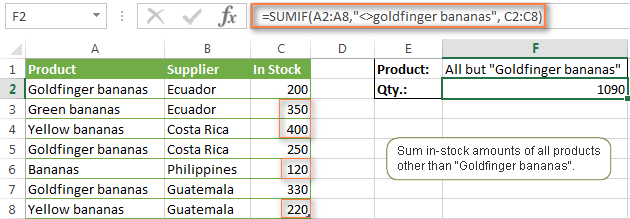Note. Like most of other Excel functions, SUMIF is case-insensitive, meaning that "<>bananas", "<>Bananas" and "<>BANANAS" will produce exactly the same result.

### Using comparison operators with cell references

If you want to take a step further and get a more universal Excel SUMIF formula, you can replace a numerical or text value in the criteria with a cell reference, like this:
`=SUMIF(A2:A8,"<>"&F1, C2:C8)`

In this case, you won't have to change the formula to conditionally sum values based on another criteria - you will simply type a new value in a referenced cell.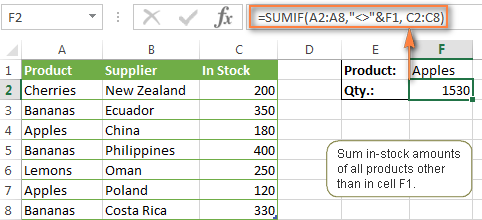Note. When you use a logical expression with a cell reference, you must use the double quotes ("") to start a text string and ampersand (&) to concatenate and finish the string off, for example "<>"&F1.

The "equal to" operator (=) can be omitted before a cell reference, therefore both of the below formulas are equivalent and correct:

Formula 1: `=SUMIF(A2:A8, "="&F1, C2:C8)`

Formula 2: `=SUMIF(A2:A8, F1, C2:C8)`

### SUMIF formulas with wildcard characters

If you aim to conditionally sum cells based on a "text" criteria and you want to sum by partial match, then you need to use wildcard characters in your SUMIF formula.

The following wildcards are available to us:

• Asterisk (*) - represents any number of characters
• Question mark (?) - represents a single character in a specific position

#### Example 1. Sum values based on partial match

Suppose, you want to sum amounts relating to all sorts of bananas. The following SUMIF formulas will work a treat:

`=SUMIF(A2:A8, "*bananas*", C2:C8)` - the criteria includes text enclosed in asterisks (*).

`=SUMIF(A2:A8, "*"&F1&"*", C2:C8)` - the criteria includes a cell reference enclosed in asterisks, please notice the use of ampersand (&) before and after a cell reference to concatenate a string.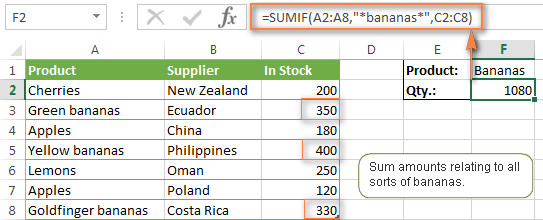If you want to count only those cells that start or end with certain text, add just one * either before or after the text:

`=SUMIF(A2:A8, "bananas*", C2:C8)` - sum values in C2:C8 if a corresponding cell in column A begins with the word "bananas".

`=SUMIF(A2:A8, "*bananas", C2:C8)` - sum values in C2:C8 if a corresponding cell in column A ends with the word "bananas".

Tip. To use wildcards with cell references, combine them with the help of the concatenation operator (&). In this example, you could also use the following formula to sum all "bananas" in stock: `=SUMIF(A2:A8, "*"&F1&"*", C2:C8)`

#### Example 2. Sum values with a given number of characters

In case you want to sum some values that are exactly 6 letters long, you would use the following formula:

`=SUMIF(A2:A8, "??????", C2:C8)`

#### Example 3. Sum cells corresponding to text values

If your worksheet contains different data types and you only want to sum cells corresponding to text values, the following SUMIF formulas will come in handy:

`=SUMIF(A2:A8,"?*", C2:C8)` - adds up values in cells C2:C8 if a corresponding cell in column A contains at least 1 symbol.

`=SUMIF(A2:A8,"*",C2:C8)` - includes seemingly empty cells that contain zero length strings returned by some other formulas, e.g. `=""`.

Both of the above formulas ignore non-text values such as errors, booleans, numbers and dates.

#### Example 4. Treat * or ? as usual characters

If you want either * or ? to be treated as a literal rather than a wildcard, then use the preceding tilde (~). For example, the following SUMIF formula will add values in cells C2:C8 if a cell in column A in the same row contains a question mark:

`=SUMIF(A2:A8, "~?", C2:C8)`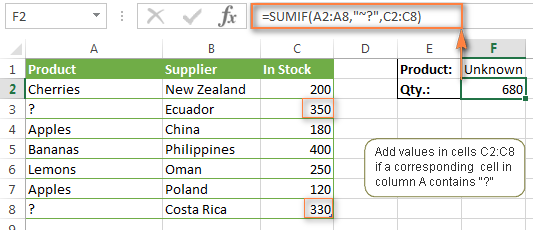### Sum the largest or smallest numbers in a range

To add the largest or smallest numbers in the range, use the SUM function together with the LARGE or SMALL function, respectively.

#### Example 1. Add a few largest / smallest numbers

If you want to sum just a few numbers, say 5, you can type them directly in the formula, like this:

`=SUM(LARGE(B1:B10,{1,2,3,4,5}))` - sum the largest 5 numbers

`=SUM(SMALL(B1:B10,{1,2,3,4,5}))` - sum the smallest 5 numbers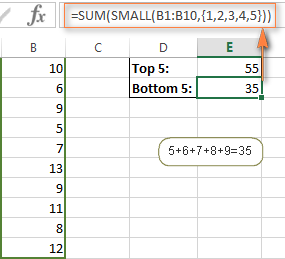Note. If there are 2 or more numbers that are tied for last place, only the first will be included. As you see, the second 9 is not added up in the above example.

#### Example 2. Add many top / bottom numbers

If you want to add up many numbers, instead of enumerating them all in the formula, you can nest the ROW and INDIRECT functions in your SUM formula. In the INDIRECT function, use row numbers that represent the number of values you want to add up. For example, the following formulas sum the top and bottom 15 numbers, respectively:

`=SUM(LARGE(B1:B50,ROW(INDIRECT("1:15"))))`

`=SUM(SMALL(B1:B50,ROW(INDIRECT("1:15"))))`

Since these are array formulas, remember to enter them in the array-way by pressing Ctrl + Shift + Enter.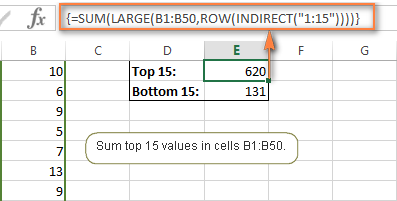#### Example 3. Sum a variable number of largest / smallest values

If you'd rather not change the formula every time you want to sum a different number of cells, you can put that number in some cell instead of entering it directly in the formula. And then, you can use the ROW and INDIRECT functions as demonstrated above and refer to a cell containing the variable, cell E1 in our case:

`=SUM(LARGE(B1:B50,ROW(INDIRECT("1:"&E1))))` - sums a variable number of top values

`=SUM(SMALL(B1:B50,ROW(INDIRECT("1:"&E1))))` - sums a variable number of bottom values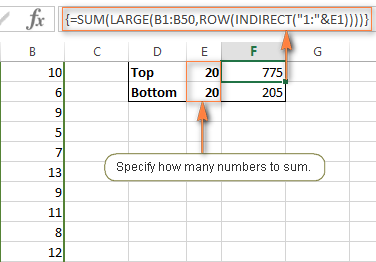Remember, these are array formulas, so you have to press Ctrl + Shift + Enter to complete them.

### How to sum cells that correspond to blank cells

If "blank" means cells that contain absolutely nothing - no formula, no zero length string returned by some other Excel function, then use "=" as the criteria, like in the following SUMIF formula:

`=SUMIF(A2:A10,"=",C2:C10)`

If "blank" includes zero length strings (for example, cells with a formula like `=""`), then use "" as the criteria:

`=SUMIF(A2:A10,"",C2:C10)`

Both of the above formulas evaluate cells in column A and if any empty cells are found, the corresponding values from column C are added.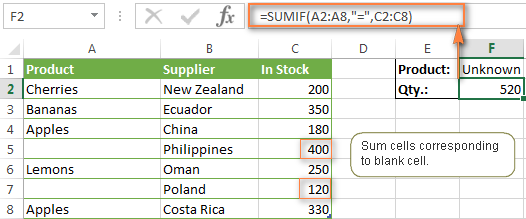### How to sum cells corresponding to non-blank cells

If you want to sum cells' values in column C when a corresponding cell in column A is not empty, use "<>" as the criteria in your SUMIF formula:

`=SUMIF(A2:A10,"<>",C2:C10)`

The above formula counts values corresponding to all non-empty cells, including zero length strings.

### How to use Excel SUMIF with dates

Generally, you use the SUMIF function to conditionally sum values based on dates in the same way as you use text and numeric criteria.

If you want to sum values corresponding to the dates that are greater than, less than or equal to the date you specify, then use the comparison operators that we've discussed a moment ago. A few Excel SUMIF formula examples follow below:

 Criteria Formula Example Description Sum values based on a certain date. `=SUMIF(B2:B9,"10/29/2014",C2:C9)` Sum values in cells C2:C9 if a corresponding date in column B is 29-Oct-2014. Sum values if a corresponding date is greater than or equal to a given date. `=SUMIF(B2:B9,">=10/29/2014",C2:C9)` Sum values in cells C2:C9 if a corresponding date in column B is greater than or equal to 29-Oct-2014. Sum values if a corresponding date is greater than a date in another cell. `=SUMIF(B2:B9,">"&F1,C2:C9)` Sum values in cells C2:C9 if a corresponding date in column B is greater than the date in F1.

In case you want to sum values based on a current date, then you have to use Excel SUMIF in combination with the TODAY() function as demonstrated below:

 Criteria Formula Example Sum values based on the current date. `=SUMIF(B2:B9, TODAY(), C2:C9)` Sum values corresponding to a prior date, i.e. before today. `=SUMIF(B2:B9, "<"&TODAY(), C2:C9)` Sum values corresponding to a future date, i.e. after today. `=SUMIF(B2:B9, ">"&TODAY(), C2:C9)` Sum values if a date occurs in a week (i.e. today + 7 days). `=SUMIF(B2:B9, "="&TODAY()+7, C2:C9)`

The screenshot below illustrates how you can use the last formula to find the total amount of all products that ship in a week.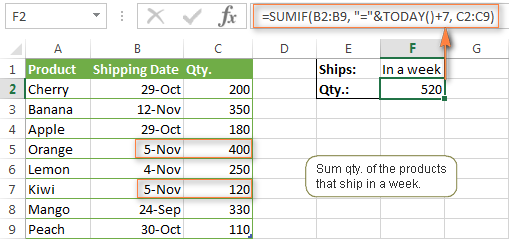#### How to sum values in a given date range

The following question is frequently asked on Excel forums and blogs - "How do I sum between two dates?"

The answer is to use a combination, or more precisely, the difference of 2 SUMIF functions. In Excel 2007 and higher, you can also use the SUMIFS function that allows multiple criteria, which is even a better option. While the latter is the subject of our next article, an example of the SUMIF formula follows below:

`=SUMIF(B2:B9, ">=10/1/2014", C2:C9) - SUMIF(B2:B9, ">=11/1/2014", C2:C9)`

This formula sums up the values in cells C2:C9 if a date in column B is between 1-Oct-2014 and 31-Oct-2014, inclusive.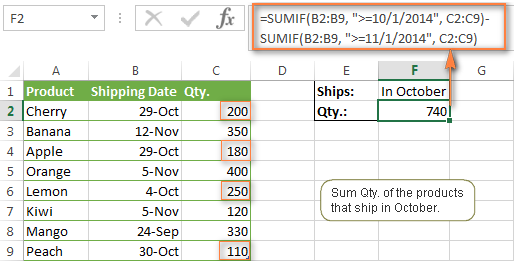This formula might seem a bit tricky at first sight, but upon a closer look, it appears quite simple. The first SUMIF function adds up all the cells in C2:C9 where the corresponding cell in column B is greater than or equal to the start date (Oct-1 in this example). Then you just have to subtract any values that fall after the end date (Oct-31), which are returned by the second SUMIF function.

### How to sum values in several columns

To understand the problem better, let's consider the following example. Suppose, you have a summary table of monthly sales. Since it was consolidated from a numbers of regional reposts, there are a few records for the same product: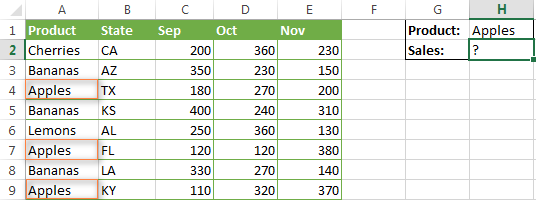So, how do you find the total of apples sold in all the states in the past three months?

As you remember, the dimensions of sum_range are determined by the dimensions of the range parameter. That is why you cannot use the formula like `=SUMIF(A2:A9, "apples", C2:E9)` because it will add up the values corresponding to "Apples" in column C only. This is not what we are looking for, right?

The most logical and simplest solution that suggests itself is to create a helper column that calculates individual sub-totals for each row and then reference that column in the sum_range criteria.

Go ahead and place a simple SUM formula in cell F2, then fill down column F: `=SUM(C2:E2)`

After that, you can write a usual SUMIF formula like this:

`=SUMIF(A2:A9, "apples", F2:F9)`
or
`=SUMIF(A2:A9, H1, F2:F9)`

In the above formulas, sum_range is exactly of the same size as range, i.e. 1 column and 8 rows, and therefore they return the correct result: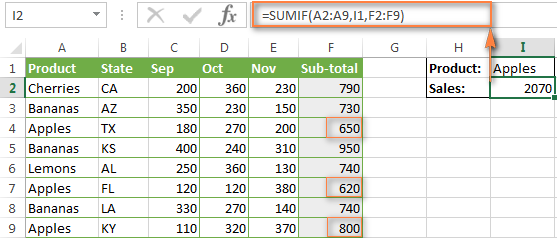If you'd rather do without a helper column, then you can write a separate SUMIF formula for each of the columns you want to sum, and then add the returned numbers using the SUM function:

`=SUM(SUMIF(A2:A9,I1,C2:C9), SUMIF(A2:A9,I1,D2:D9), SUMIF(A2:A9,I1,E2:E9))`

Another way is using a more complex array formula (don't forget to press Ctrl + Shift + Enter):
`{=SUM((C2:C9+D2:D9+E2:E9)*(--(A2:A9=I1)))}`

Both of the above formulas will return 2070 in our case.

## Why my SUMIF formula is not working?

There could be several reasons why Excel SUMIF is not working for you. Sometimes, your formula does not return what you expect only because the data type in a cell or in some argument isn't suited for the SUMIF function. So, here is a list of things to check.

#### 1. The range and sum_range parameters should be ranges, not arrays

The first (range) and third (sum_range) parameters of your SUMIF formula must always be a range reference like A1:A10. If you try to pass in anything else, for example an array like {1,2,3}, Excel with throw an error message.

Correct formula: `=SUMIF(A1:A3, "flower", C1:C3)`

Wrong formula: `=SUMIF({1,2,3}, "flower", C1:C3)`

#### 2. How to sum values from other sheets or workbooks

As almost any other Excel function, SUMIF can reference other sheets and workbooks, provided they are currently open.

For example, the following formula will sum the values in cells F2:F9 in Sheet 1 of Book 1 if a corresponding cell in column A if the same sheet contains "apples":

`=SUMIF([Book1.xlsx]Sheet1!\$A\$2:\$A\$9,"apples",[Book1.xlsx]Sheet1!\$F\$2:\$F\$9)`

However, this formula won't work as soon as Book 1 is closed. This happens because the ranges referenced by SUMIF formulas in closed workbooks get de-referenced into arrays, and since no arrays are allowed in the range and sum_range arguments, a SUMIF formula will throw a #VALUE! error.

#### 3. To avoid problems, make sure range and sum_range are of the same size

As noted in the beginning of this tutorial, in modern versions of Microsoft Excel, the range and sum_range parameters does not have to be equally sized. In Excel 2000 and older, unequally sized range and sum_range can cause problems. However, even in the most recent versions of Excel 2010 and Excel 2016, complex SUMIF formulas where sum_range has less rows and/or columns than range are capricious. That is why it's considered a good practice to always have the range and sum_range arguments of the same size and shape.

#### 4. How to make your SUMIF formulas work faster

If you've populated your workbook with complex SUMIF formulas that slow down your Excel, check out this article and learn how to make them work faster. The article was written quite long ago, so don't be surprised by the calculation time. Their recommended approaches and formula examples are still actual and brilliant!

The Excel SUMIF examples described in this tutorial only touch on some of the basic usages of this function. In the next article, we'll investigate advanced formulas that harness the real power of SUMIF and SUMIFS and let you sum by multiple criteria. Please stay tuned and thank you for reading!

### 234 Responses to "How to use SUMIF in Excel - formula examples to conditionally sum cells"

1.NAZAR says:

IN A FROM 1-31 IS DATE N IN B C D ... IS THE NAME OF THE PERSON
IF B IS PRESENT IN DAY 1 I HAVE TYPE Y AND IF NOT THEN I HAVE TYPE N. NOW I WANTS TO SUM THE HOW MANY DAYS B OR C OR D WAS ABSENT OR PRESENT HOW CAN I GET THE RESULT IN LAST CELL/COLUMN NO IN 32 NO CELL

2.NAZAR says:

AND IS THERE ANY OPTION PROCESS ETC THROUGH EXCEL CAN FLASH OR REMIND 15-30 MINUTES BEFORE ANY MEETING ETC

3.Fazal says:

Hi how can i calculate sum of range if the range has to be given by user.

If i give 5 in a cell and i need sum of range from a1 to a5 in my result cell B2

And if i give 7 result should be sum of a1 to a7

4.Marvin says:

I have a column of finishes, I only want to count the total finishes below 41.

5.JOLLY. says:

please how can i have continous additions of numbers each time new numbers is imputed in excel

6.Iwan says:

Hi,

Thanks for this wonderful step-by-step teaching about Excel. I find it very useful.

I have a problem regarding SUMIF issue. Let me explain.

=SUMIF(Inc!\$M\$1:\$M\$1000, ">=1/2/16", Inc!\$J\$1:\$J\$1000)- SUMIF(Inc!\$M\$1:\$M955, ">=7/2/16", Inc!\$J\$1:\$J\$1000)

How can I change ">=1/2/16" and ">=7/2/16" to a cell number like B2 or C3 that contains the particular date like 1/2/16. So, I have made 2 cells that have 1/2/16, and the other one is 7/2/16. Instead of having to input the date manually in the formula, I want to input the cell number that already has the date information in it so I can just copy it down.

Thanks

Iwan

7.Rea Sasseville says:

I want to do a monthly ytd total on a spreadsheet with the months in columns. I have a ytd column and am trying to put in the sumif forumla taking the months Jan - Dec and giving them a value of 1 - 12. I then, in the cell immediately above my formula, have the current month's value. My sumif function is =Sumif(h5:s5,"<=v8",h9:s9) my calculation would be then that it should add all of the columns numbers 1 - 11 as that is the number displaying in v8 and not add the number in the 12 column. However it is adding it. What am I missing?

8.Mohamed Azhar says:

Dear
Greetings..!

I'm trying to calculate SUMIF function but i do not know how to calculate and getting my answer but i would expecting you guys will solve my problem in excel.

i have an excel sheet that contain in Column (A) Date as (2-12-2016 9:37 PM) in Column (B) Names Like (Adam, John, Wiki) in Column (C) an Amount such as 100, 200, 500 in Column (D) Should be My Answer that's are follows

if in Column (A) Date "1-1-2016 to 31-1-2016" and in Column (B) "Adam" then Calculate the amount in Column (C) "100" and show the Addition in Column (D)

i tried this but i'm fail

Kindly Help me

9.Jimmy says:

I can't seem to get this to work. Is this formula legal or possible?

=SUMIF('ITSC-0101S-01:ITSC-0909S-01'!E3, B3, 'ITSC-0101S-01:ITSC-0909S-01'!B3)

What is supposed to do is look at cell E3 across multiple sheets and IF it matches cell B3 on the formula sheet then add the corresponding cell B3s together.

10.Ccil says:

Hello,

Here is my questions with the example

I have in F2 = "Apples/Bananas"
I need my formula to be =sumif(A2:A8,F2,C2:C8)

In F2 I can have whatever sign or space that make this formula works.
I need this formula to add Apples and Bananas.

Any thought or help ?

C.

11.Sha says:

What a great Blog!

Im not great at excel but your explanations are so simple and easy to understand!!!

I do have a question though :-p I am currently using you're SUMIF formula for specific text.

=SUMIF('Main Tracker'!G7:G30,"*GBCDF*",'Main Tracker'!T7:T30)

I was wondering can you also add a date range to that formula so that it totals up everything with "GBCDF" within a certain date range.

12.DallasJewel says:

This blog is phenomenal. I am stumped. I have an account number for my customers is column A and Column B lists the sale amount. I would like to have column c aggregate the sales for each customer. Some customers have 1 sale each month some have 10. How do I get a running total for customers in column c

•Svetlana Cheusheva (Ablebits.com Team) says:

Hi DallasJewel,

Is my understanding correct that there can be several occurrences of the same account number in column A if a customer has more than 1 sale? If so, you can use this formula:
=SUMIF(A:A, A1, B:B)

If you are looking for something different, please clarify.

13.Darren Haste says:

Hi, Great blog and I'm hoping you can help?

I have a large sheet called 'Errors' (11 columns) and in Column A I have employee names who have recorded till errors over 2016, so their names appear multiple times down the list and the value of the error short/over appears in Column J.

In a separate tab I have the full list of employee names, so for example A1 is Adam. In B1 I'm trying to create a formula that will return all shortages created by Adam in 2016 by looking at the other sheet (A1:K4000. I've been trying something like =VLOOKUP(A1,ErrorsA1:K4000,10,"<0) but it returns a #value error.

Any help would be greatly appreciated.

Darren

14.lily says:

how to sum up c1:c50 when b1:b50 is not o or c or oc?

15.tohan says:

Great article! I thought I knew SUMIF but I learnt a lot of things there.

Similarly to sum-range indicating only the upperleft-most cell, it would seem that range actually indicates only the upperright-most cell is that correct ?

I stumbled upon a spreadsheet that I couldn't understand how, was giving the correct result, basically instead of =SUMIF(\$B\$2:\$B\$10,\$H\$2:\$H\$10,\$D\$2:\$D:\$10) it read =SUMIF(\$A\$2:\$B\$10,\$H\$2:\$H\$10,\$C\$2:\$C:\$10)
I now understand the shift to column C in 'sum_range' as 'range' was 2 columns wide, but I wanted to confirm the behavior of 'range'.
Thanks!

16.AKASH says:

hi, just suppose we have 2 list. in first we have customers account and pending amount. in second list of some customers with account who have paid partial of full amount. then how to find net amount? if list are large and we cant sort them..

17.Steve says:

I am trying to use the sumif function to sum a series of job #'s that are specific to salespeople. I am exporting this list out of our accounting software & I noticed that the job #'s are actually labeled as "text" in my worksheet. What would my criteria be for the following:

Job Billed to Date

2074-14 4,306.11
2999-17 0.00
4000-17 0.00
4001-17 0.00
4002-17 0.00
4003-17 0.00
4004-17 0.00
4005-17 0.00
4006-17 0.00
4070-16 18,462.00
4076-16 10,133.00
4082-16 7,940.00
4083-16 7,610.00
4091-16 7,895.00
4092-16 5,925.00
4093-16 7,510.00
4094-16 0.00
4095-16 15,863.00
4096-16 7,876.00
4097-16 7,200.00
4098-16 18,360.00
4101-16 5,450.00
4102-16 7,500.00
4103-16 12,100.00
4104-16 0.00
4105-15 9,510.00
4105-16 0.00
4106-16 2,950.00
4107-16 7,754.00
4108-16 0.00
4999-17 0.00
5000-16 53,529.00
5004-16 22,683.00
5005-16 1,419,164.00
5007-16 30,299.00
5008-16 0.00
5011-16 0.00
5013-16 0.00
5014-16 0.00
5015-15 111,403.52
5015-16 0.00
5016-16 0.00
5017-16 0.00
5018-16 0.00
5024-15 57,166.00
5999-17 0.00

Column "A" is the job#, column "B" is the billed amount. I am trying to sum the sales for column "A", which each range represents a salesperson. Ex., series 2000-2999 jobs = Bob, series 4000-4999 = Joe & series 5000-5999 = Marie.

Here is the formula that I am trying to use, but it is not working. Any ideas? Thanks

=SUMIF('Sep 16'!A2:A1048576,">2000,<2999-99",'Sep 16'!B2:B1048576)

•tohan says:

You could do:
=SUMIF('Sep 16'!A2:A1048576,"2*",'Sep 16'!B2:B1048576) to sum up all job# starting with 2 for instance (repeat accordingly for 3, 4 and 5s or build up a formula like so: C2&"*" for your criteria)

18.Ashley says:

Hi,

I am trying to autosum a total column in an invoice table spreadsheet in which it includes the data and the price visited per site. I have written a formula in the date column like such: =IF([@Date]"",167, 0). Hence if there is a date entered it will auto populate 167 in the Price column in the table, if it does not have a date inserted it just '\$ - ". But when I try to SUM or IFSUM (=SUMIF([Date], "", [Price]) formulas it rather leaves a "\$ - "or has the #### error. I have tried SUMPRODUCT, SUM, SUMIF, and SUMIFS formulas but cannot get it to total sum the columns that have a price compared to not having anything. Please help!

19.Rohit Saluja says:

Hi,
Is it possible to add a mathematical condition in Sumif formula. Please refer below example

A
1
2
3
4
5
6
7

Can I add a condition in Sumif such that add only those numbers which are divisible by 3.

20.Francis says:

Hi there,

I have a question on sumif and sumifs function. I have a list of data showing contracts names that took place over 2012-2014 for a specific client. I want to set up a summary tab where there are drop downs for contract name and years. so if I were to select 2014 and it'll give me a total figure of how much was made in that year. If I were to select specific contract name for 2014, it'll give me how much was made on that contract in 2014. Also if I was to select all years and all contracts, it'll give me an overall total.

Would anyone happen to know how I would go about doing that please??

thanks

21.Jyothi Prakash says:

Hello

I have cells having letters or numbers and i want to add those cells if they have number and if the cell has letter should be discarded. How to do this?

22.GEBRETSADIK ABRAHA says:

THANK YOU FOR SHARING US KEEP UP.

23.Bethany Harris says:

Hello,
I am working on an excel sheet for work and I need to create a cell that will add up how many rows I have that are a certain color and display that number of rows in the cell. I also have one that needs to add up the number of rows based on color AND a specific word in a cell within that row. If there a formula I can use to to this? I can only find ones that do one or the other.

Ex: Some rows are green, some are yellow and others are orange. Green represents a kind of home and yellow a different and orange a third kind of home. The yellow and orange ones are only color specific and I have a cell at the top that needs to add up the number of yellow and orange rows I have and give a number. The green will change based on a column that will describe if this home is open to build or already being built. I need a formula that will only find the green lines with this specific text in the column and add those up and give a number value in the cell of how many green cells there are with this text. (I hope that helps)

24.Brett Cilton says:

Hi,

I am working on a cash flow. I'm looking to create a formula which basically read's: If (A1) = (B1) then add (C1) to (D1).

I hope this makes sense.

25.Dean says:

Hi, hope you can help me to find the best formula for my need.
I would like to make revenue calculation of several customers.
there is a commission that is changing between some of them - for example
1. should be amount - 20%
2. should be amount + 5%
3. no extra commission

I have used =sum with sumif for each one that needs extra commission change but than I don't know how to add in all the rest that don't need any modification without the sumif...my formula:
=sum(SUMIF(B96,"customer1",E96)*\$L\$3)+(sumif(B96,"customer2",E96)*\$L\$4)+(sumif(B96,"customer3",E96))+(sumif(B96,"customer4",E96))-G96

*note that I added customer 3 and 4 without any modification just so they are counted...but I need something generic for any other partner than 1 and 2
any idea?

Thanks!
Dean

26.Johncy says:

Hi,

How to sum cells which contains numbers and text either the numbers are in the beginning or at the end? I don't wanna remove the text/number or don't want to separate the text and the number.

27.Doug says:

Hello. I'm trying to write a sumifs formula for multiple conditions in which the referenced criteria cells contain formulas and display the results. For example, instead of summing all the cells in a column where the value >=2, I want to sum all the cells where the value is >= a referenced cell that has a formula in it that equals 2. In older versions of Excel I did this with the Conditional Sum Wizard, but I can't seem to get it to work with Sumifs. Can you help.

Thanks so much!

28.Gareth says:

Hi,
I am trying to write a formula to add up the values in a previous year based on the equivalent amount of recorded days this year to show a Year on Year variance.

I have a support worksheet that adds up the amount of days / cells that has had data inserted in this year to produce a number. (for example 18 days)

I have a list of values separated by days from last year and i want to return a value based on 18 days worth of data from that year.

•Natalia Sharashova (Ablebits.com Team) says:

Hello, Gareth,
I'm afraid it's a bit difficult to assist you without some specific data. You can read this part of the article more attentively to sum up the dates, otherwise, please, give us more details on your task.

29.Alex says:

I have multiple sheets with the same data and I am able to sum the corresponding data with =SUM(Leuzinger1:Sheet2!C3). Now I want to only sum the value in C3 from a sheet if the value in A1 of that sheet is 1. Can I use sumif? Basically, I need to select all the sheets where A1 == 1 and then sum the values of c3. Thanks for any help!

30.Adi Arpadzic says:

Hi, i have problem with SUMIF function. My criteria is acrticle code (ex. 031285), and SUMIF bring me result from 031285 and 31285 so it is incorrect then. How to force SUMIF to bring only exactly code article? Thanks!

•Adi Arpadzic says:

i need force SUMIF to use my zeros in article code to criteria when finding exact value from that article code.

31.Waqas Ali says:

Hello

I am using my sumifs with 2 criteria
Sumif(a2:a7, d2:d7, >8years, e2:e7, "m")

The age (d2:d7)I am converting using datedif

The formula works up to 9years 11 moths, as soon as the age is over 10 years, it does not add numbers in a2:a7

Any help would be appreciated.
Thanks

32.Brajesh says:

Hai

I have three columns e.g.

USA 10.08.2017 10.54
India 10.08.2017 9.45
USA 11.08.2017 6.54
USA 12.08.2017 2.98
India 12.08.2017 1.65

Now if destination repeats then sum the current value plus value of same destination before 48 hours from current date

Can you help.

Thanks so much!

33.Richard says:

Can you use the SUMIF to sort by date range and cell info?

34.Asil Zada says:

MLot# CN Customer Colour Fabric Gry Wt Rate Str Print
CL-10531 Bal ES Apparel Navy HTR S/J 351.25 95
CL-15558 Dyd Mass Apparels & Fabrics (PVT) Ltd. H/Grey 2Flc 981.00 50
CL-16455 Bal Crown Textile Boardex 3Flc 622.00
CL-16664 Dyd Salman Industries Wind Chime 2Flc 707.05 Yes
CL-16732 Dyd Salman Industries Peach Whip 2Flc 711.70 Yes Yes
CL-16900 Dyd Salman Industries H/Grey 2Flc 526.70 90 Yes
CL-16901 Fnsh Salman Industries Plane Moul 2Flc 974.40 Yes Yes
CL-16913 Dyd Pelikan Knitwear Blue Flot F/T 579.90 175 Yes
CL-16990 Fnsh Salman Industries H/Grey 2Flc 978.95 90 Yes Yes
CL-17058 Dyd M. R. Export H/Grey 3Flc 972.50 Yes
CL-17192 Dyd Pelikan Knitwear Blue Flot F/T 742.00 175 Yes
CL-17193 Dyd Zubisma Apparel Navy 3Flc 473.20 Yes Yes
CL-17244 Dyd Pelikan Knitwear Black 3Flc 440.25 160 Yes
CL-17250 Dyd M. R. Export Mid Night Navy 2Flc 794.35 Yes
CL-17278 Dyd Pelikan Knitwear White 2Flc 1002.05 Yes Yes
CL-17321 Dyd Pelikan Knitwear Charcoal 2Flc 315.00 140 Yes
CL-17339 Dyd M. R. Export H/Bleach 3Flc 954.95 Yes

Table[CN]="Dyd" And Table[Str]="Yes" And Table[Print]="Yes"
Then Sum of Table[Gry Wt] * 20

Result should be =
711.7 * 20 + 473.2 * 20 + 1002.05 * 20 = 43,739

35.Jim says:

Cell C5 displays the sum of C1:C4
If I want Cell C6 to show the total in excess of B5
but if C5 is less than B5 I want it to show 0

How can I do this?

36.Sue says:

My spreadsheet has one cell (A1) that contains the "pot" of money. One column (cell A2) is for amounts drawn against it with another column (C2) having a balance. So the formula in C2 is =SUM(A1-A2).

Another column is what was “actually spent”, so if I put a value in that one, I would like to have C2 deduct that instead of the original amount drawn. This column will only have a value if what was actually spent is more or less than original amount.

I'm looking for a formula something like "=SUM(A1-A2) unless B2 has a value then use that instead of A2”?

37.Suraj Kc says:

I need SUMIF FORMULA for certain 'Entry Name' along with seperation of Date from 1 to 30.
Example
A NAME HOURS
1.9.2017 ABC 2
1.9.2017 ABC 3
1.9.2017 XYZ 2
2.9.2017 XYZ 1
2.9.2017 ABC 2
3.9.2017

How can I get total hours of 1.9.2017 for ABC and XYZ seperately?

DATE ABC XYZ
1.9.2017 5 2
2.9.2017 2 3

I need total hours for ABC and XYZ on basis of dates and name entered.

Email: surajkc938@gmail.com

Thank You!!

38.Darin says:

Thank you in advance for any assistance.
I'm trying to SUMIF the amounts in H if they occurred on a given day.

Formula is =SUMIF(TEXT(\$A2:\$A36, "mdyyyy"), TEXT(G41, "mdyyyy"), \$H2:\$H36)
A2= 9/9/2017 (cell formatted as date)
H2= \$2,201.88 (cell formatted as currency)
G41= 9/9/2017 (cell formatted as date)

When I view the calculation via fx, everything looks fine, but the result is 0.

Thank you again for any insight.

Best,
Darin

39.Ahamed Wazeer says:

Hello

How can able to calculate Commission as per ton
conditions are following

less than equal 60 ton will pay 100\$ if more than 60ton additional tons will pay in 150\$

how to formulate for this case which function i need to use

40.Shameer says:

I have invoice no in one column its may repeating depend upon how many items in a invoice, i need total value of item that is present in same invoice no

41.aas1354 says:

Hello everyone,
I have a formula which is like this

=COUNTIFS('sheet1 '!W:W,">0",'sheet1 '!A:A,"Truck")

which is count all data in column W (sheet1) larger than 0, which are called Truck in column A.

Now, I want this formula to read the same information but by consider a range of dates in between 1st of October to 15th (I have dates in Column B).
Any input is greatly appreciate.
Thanks

42.Evangeline Hurter says:

Working on a school fundraiser. We offer items for \$3 each or 5 for \$10. Is there a formula for determining not only the total sold but also calculating the amount of monies that should have been collected?
Thanks

•Gennady Terekhov (Ablebits.com Team) says:

Hello,
For me to understand the problem better, please send me a small sample workbook with your source data and the result you expect to get to support@ablebits.com. Please don't worry if you have confidential information there, we never disclose the data we get from our customers and delete it as soon as the problem is resolved.

43.Sharon says:

I have a Y / No column. I want to know how many Yes and how many No. I'm sure this is very simple, that I just can't break out of the simplicity of it.

•Gennady Terekhov (Ablebits.com Team) says:

Hello,

="Yes - "&COUNTIF(A:A,"Y")&" No - "&COUNTIF(A:A,"No")

44.Sammy says:

How can I use the sum (small) Function when my values are in non contiguous cells and not in a range?

•Gennady Terekhov (Ablebits.com Team) says:

Hello,

=SUM(SMALL((B1,B3,B7,B9,B10),{1,2,3}))

45.Ahmad says:

i want to check master SKU primary volume sale distributor type wise by latest date. format mention below.

Date Master SKU Distributor Type Primary Volume
12/5/2017 Olper 200ml Hyperstar - ICA 365
12/5/2017 Olper 200ml Makro/Metro –ICA 480
12/6/2017 Olper 200ml Hyperstar - ICA 72
12/7/2017 Olper 200ml Makro/Metro –ICA 38
12/5/2017 OMUNG 200 ML Hyperstar - ICA 178
12/5/2017 OMUNG 200 ML Makro/Metro –ICA 120
12/6/2017 OMUNG 200 ML Hyperstar - ICA 58
12/7/2017 OMUNG 200 ML Makro/Metro –ICA 48

•Gennady Terekhov (Ablebits.com Team) says:

Hello,
For me to understand the problem better, please send me a small sample workbook with your source data and the result you expect to get to support@ablebits.com.

46.Siddique paniwala says:

How to use sum or sumifs in this condition

If value is is start to 1 and end to 130 and then restart to 1

•Gennady Terekhov (Ablebits.com Team) says:

Hello,
For me to understand the problem better, please send me a small sample workbook with your source data and the result you expect to get to support@ablebits.com. Please don't worry if you have confidential information there, we never disclose the data we get from our customers and delete it as soon as the problem is resolved.

47.Alex says:

Hi!
I am working on 2 sheets, sheet 1 & 2 which I re-named GENERAL and SUMMARY.
The GENERAL sheet is where I do my in & out of materials and the SUMMARY sheet is where I get the balance quantity of the materials.
e.g. material code on column H and quantity on column K of the GENERAL sheet, then material code on column B and balance quantity on column E of the SUMMARY sheet. I tried =SUMIF(GENERAL!H4:\$K4:B4, 3331, GENERAL!B4:\$E4, 3331) but failed. Kindly help

48.ajay says:

Invoice date at row number 1 is less than October 2014.Invoice Date should be less than Current Date and greater than October 2014.Error in Excel Sheet Data.

49.Sajil says:

If Cell A says "POS" i need to get 3% of cell B & C at Cell D,
If Cell A Says "NAG" i need to get 5% of Cell B & C at Cell D

50.Musaddique Anwar says:

Hi! Sir
I am from Malegaon. I am businessman.
I watched all videos on your youtube channel about excel. All videos are very useful but I have one problem.

The problem is.........
CONSIDER:

this is [ ] first column in excel.
this is ( ) second column in excel.

In this [ ] type number.
In this ( ) number should come automatically.

In this [ ] column 0 to 80 is capacity and after 80 starts from 1.

 (45) this is 0+45=45
 (35) this is 80-45=35
 (25) this is 0+25=25
 (25) this is 50-25=25
 (40) this is 80-50+10=40
 (50) this is 60-10=50
 (30) this is 80-60+10=30
 (35) this is 45-10=35
 (30) this is 75-45=30
 (20) this is 80-75+15=20
 (35) this is 50-15=35
 (50) this is 80-50+20=50

51.Chris says:

Hello!

I am looking to do a Sumifs based on the following conditions:
Sum = "Good" Column
Part = "A"
Step = Smallest value for Part "A" assuming this can change depending on data pull.

PART STEP GOOD
A 10 10
A 10 10
B 30 5
B 30 10
B 50 15
A 20 10
B 60 8
C 20 6
C 40 10
C 50 20
A 30 30
B 30 40
C 60 20
A 30 15

Thank you, any suggestions would be much appreciated!

•Doug says:

Chris:
Where the data is structured as your sample and is in F36:F49,D36:D49 and E36:E49 enter the "Part" in I36 and the "Step" in I37, then in I38 enter the formula:
=SUMIFS(F36:F49,D36:D49,I36,E36:E49,I37)
You can then enter the step and part you're interested in seeing in their respective cells and the Good will be displayed in I38.
You'll probably want to enter their labels in H36, H37 and H38.

52.Mohammad Yamin says:

I want to apply a simple IF formula

A1-A2=A3
10-12=0
10-8=0
IF the result is <0 A3 should show 0 else the actual value.

For example A1 value is 10 and A2 value is 12 and I want result in A3 only 0 if the result is <0.

53.Shabbir says:

I Want Help On following
Tax Slab Rate of Tax
- 400,000 0
400,001 800,000 1,000
800,001 1,200,000 2,000
1,200,001 2,400,000 5% of exceeding of 1,200,000
2,400,001 4,800,000 10% of exceeding of 2,400,000 & 60,000
4,800,001 onwards 15% of exceeding of 4,800,000 & 300,000

Taxable Income Is 3,880,000 Per year
And Tax Liability 208,000 Per Yeat
and i used the Following Formula for calculation
=IF(C11<=B4,0,IF(C11<=A5,1000,IF(C11<=A6,2000,IF(C11<=A7,(C11-B6)*0.05,IF(C11<=A8,(C11-B7)*0.1+60000,IF(C11<=A9,(C11-B8)*0.15+300000))))))

54.maharshi says:

hi,

i am maintaining invertory in excel, by the current date
opening stock has to come with the total of previous for specific item.
please guide the formula. my formate is as follows

item Description 19-Jun-18 20-Jun-18 21-Jun-18 22-Jun-18
Item No.1
Item No.2

55.Bill Hawkes says:

How can i use Sumif statement with the condition or criteria is a highlighted cell? looking to pull cost based on Green, Yellow, or Red Cells. any help would be great.

56.Phil says:

I want to use several Sumifs and all work except for the following.

In column B there will be a list of numbers and I want the calculation to exclude all numbers that end with the number 2, is this possible? or the reverse add up all the numbers that don't end with the number 2.

Phil

57.Peter says:

So I'm running into some issues with a compound SUMIF formula.

=SUMIF(AND(A8:A200,G8:K200,">"&Q1,G8:K200,""&0)

The main issue is that there are missing values for the lines that have a 0 in the "count" column as the information is being pulled from another table where "Count" is compiled using a COUNTIF formula. This being the case, these rows have the #N/A value plugged in if the count column shows a 0 which prevents the data from calculating. Because of this, I am hoping someone may be able to help me out and suggest a way for me to add up the dollar values for any dates that appear in a column that is between Days 1 and 2.

Essentially I need it to do the following:

1. Check columns G for any dates within a 1week timeframe
2. add up the values that have a quantity of 1 or more.

does anyone have any suggestions?

58.G says:

Substantially important point below, don't think I've seen this nuance pointed out anywhere else!

"The point is that Microsoft Excel does not rely on the user's ability to provide matching range and sum_range parameters, so to avoid possible inconsistency issues and prevent errors, it determines the sum range on its own in the following way. It takes the top left cell in the sum_range argument as the beginning cell (C2 in our Excel SUMIF example), and then includes as many columns and rows as contained in the range argument (in our case, it's 1 column x 7 rows, i.e. C2:C8)."

59.DB says:

I'm trying to develop a mileage tracking sheet for both personal and business entries. Column A is the odometer when I arrive at a certain location. Column B will have a "Y" if the mileage is personal in that row. Column C is the total miles driven since the last stop if it is for business, no "Y" in column B for that row. Column D is the total miles driven since the last stop if it is for personal, "Y" in the B column for that row. Cell H2 contains a running total of business miles for the curent month. Cell H4 contains a running total of personal miles for the current month. I can't figure out how to subtract, for example, cell A5 from cell A6 using the SUMIF or SUMIFS function. I think I can write a formula for a running total for personal and business miles driven, but if you can suggest a formula I would appreciate it. Do I need to write a formula or include in the formula where to place the total miles driven for any certain row in either column C or D? Meaning for business miles driven on a single drive, no "Y" in column B for that row, and place that number in column C for that row and leave column D for that row blank. As you can tell, I'm struggling with these formulas. Thank you in advance for your help. DB

60.Gerry says:

I wish to be able to allow a cell ( D6 )\$ amount to be included in the column ( D1 to D20 )total if the next cell ( E6 )is marked with a check symbol ( wingdings 2 ). It said cell ( E6 ) is marked with an x ( wingdings 2 ), then the cell ( D6 ) with the \$ is not included in total. And so on down the page for my monthly bills accounting.

61.Amy Yee says:

Hi, how to combine both formulas in one cell?
=SUM(H31,I31,J31)
=IF(H31<I31,I31)+If(H31<J31,J31)
I need to sum 3 coloums (H,I,J) but if I & J have values then H will need to subtract.
Thanks & regards,
Amy

62.Ahmed says:

I have a tracking table for tracking Design request enquires.
i need to track on MONTHLY basis total number of Design Requests with Status (open / Close) and Overdue (Yes / No)

63.Michelle says:

Just what I needed. Thanks for the detailed explanation.

64.Cindy says:

Thank you so much for this site. I was able to finally figure out the function that I needed and how to write the different parts.

65.Sohaib says:

Thankyou so much for being help us. but here i want to ask how we can copy text statement from one sheet to another sheet so that if we changed in master sheet the change could appear in other sheet automatically.

66.Mazen says:

Hello there,

I need a formula to do the following...
if column D contains today's date, and column F contains "apple" then SUM column E.

67.Vicki says:

Thanks for posting this! Great information and clearly presented!

68.HAREESH says:

Sir I need a help.
I want to addition 2 column with a condition ie
need calculation in U4,condition is that if column no "N4" is >= column No F4, need 0 if not, need original amount while adding N4+T4

•andy says:

hi,i am struggling with calculating data.
how to calculate if range of specified text is met then sum range greater than 0 in one cell.
eg. IF(A2:A50,"abc") THEN SUMIF(F2:J15,"<0")

Thank you for looking in my post.

69.HAREESH says:

Sir I need a help.
I want to addition 2 column with a condition ie
need calculation in U4,condition is that if column no "N4" is >= column No F4, need 0 if not, need original amount while adding N4+T4

70.Quentin says:

hi i dont know if this is the right place to ask but. i have some columns that has either 1 or 0 in them and i need to add up the 1 up to the first 0 and no further.problem is there may be hundreds of 0 in the same column. and the same for 1. so my question is how can i add up all the 1 up to the first 0 and nothing else. ive been doing it manually but it is annoying plus the chance for error is bigger.

71.Nagaraju says:

72.anil babu says: